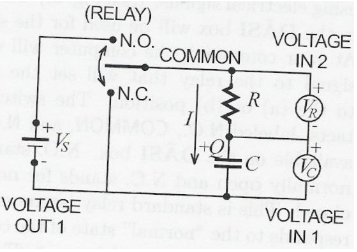# Problem: Which of the following statements are TRUE about the circuit shown below?1. When the current I is positive, the capacitor charge Q is decreasing.2. When the current I is positive, the measured VOLTAGE IN 2 will be negative.3. When the capacitor charge Q is positive, the measured VOLTAGE IN 1 is positive.4. The capacitor charge Q exponentially decays to zero when the RELAY is thrown to position N.O.5. The transient currents that flow in this experiment decay exponentially to zero.

###### FREE Expert Solution

1. When the current is positive, the capacitor will exponentially decay to zero. Hence NOT True.

91% (112 ratings)###### Problem Details

Which of the following statements are TRUE about the circuit shown below?1. When the current I is positive, the capacitor charge Q is decreasing.

2. When the current I is positive, the measured VOLTAGE IN 2 will be negative.

3. When the capacitor charge Q is positive, the measured VOLTAGE IN 1 is positive.

4. The capacitor charge Q exponentially decays to zero when the RELAY is thrown to position N.O.

5. The transient currents that flow in this experiment decay exponentially to zero.